#### Flip-Flops, Physics tutorial

Introduction:

The combinational logic circuits operate firmly in accordance with their truth table. Though, there are logic circuits that have feedback path and operation of which is not firmly stated by their truth tables.

Such circuits operate in a different way for given input condition depending on previous input sequence applied to circuit. Such circuits are called as sequential logic circuits. These circuits have memory element also. As well as logic gates, the computer needs memory element. Simplest memory element is a flip-flop. It has two stable states and remains in any one of these two stable states until triggered in other state. Quite frequently flip-flop is also called as a latch.

RS Flip-flop:

Most basic flip-flop circuit is built using two NAND gates or two NOR gates. In NAND gate flip-flop, two NAND gates are cross-coupled. It has two latched outputs Q and Q‾. It has two inputs: SET (S)' and RESET (R) or CLEAR (C). Input names mean their actions also. For input names such a flip-flop is called as RS flip-flop.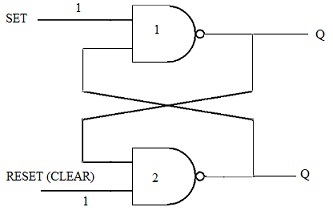Now understand working of RS flip-flop. Both inputs, SET and RESET, are kept HIGH, i.e. at logic 1. In beginning, let us say S = R = 1. With outputs Q = 0 and Q‾ = 1, NAND-1 has inputs 1 and 1 therefore Q = 0, and NAND-2 has inputs 1 and 0, therefore Q = 1.

These outputs are latched or stuck with each other and continue to be latched until input conditions are changed. Second possibility with S = R = 1 is when Q= 1 and Q‾ = 0. NAND-1 will have 1 and 0 inputs giving Q= 1. Similarly NAND-2 will have 1 and 1 inputs giving Q‾ = 0. The two outputs are latched together and they will carry on to be latched until input conditions are changed. S and R both high means two sets of possible outputs remains in last state indefinitely due to internal latching action. Therefore, high S and a high R provide us inactive state; circuit stores or remembers. When we want to change flip-flop output one of the inputs will be pulsed LOW (i.e. logic 0).

Setting the Flip-flop:

Let us say that SET is momentarily pulsed LOW (i.e. S = 0 for a moment) while RESET continues to be 1. Now if Q= 0 and Q‾ = 1 before occurrence of LOW pulse at SET, Q goes 1 which in turn forces Q to a 0. Therefore when SET returns to 1, NAND- 1 output remains HIGH which in turn keeps NAND-2 output at 0.

If before, the application of SET pulse, Q= 1 and Q‾ = 0, then LOW pulse at SET won't change anything as Q‾ = 0 is already keeping NAND-1 output to 1. Therefore, when SET returns to 1, outputs are still Q= 1 and Q‾ = 0. Therefore a LOW on SET input will always cause flip-flop to end up in Q = 1 state. Therefore, this operation is known as setting flip-flop and Q = 1 state is called as SET state.

Resetting or Clearing Flip-flop:

SET is kept at 1 and RESET is momentarily pulsed LOW (i.e. 0). Let us say that before pulse, Q= 0 and Q‾ = 1. As Q= 0 is already keeping NAND-2 output at 1, thus application of LOW pulse at RESET won't change situation. Though, if before application of LOW pulse, Q= 1 and Q‾ = 0 , then the LOW pulse at RESET will give NAND-2 output as 1, which in turn forces NAND-1 output to a 0. Therefore a LOW at RESET always ends up in Q= 0. This operation is known as clearing or resetting operation. And Q= 0 state is called as CLEAR (or RESET) state. When SET and CLEAR are concurrently pulsed LOW, it generates 1 at both the outputs. There is a race to come to a 1 state. This is the undesired state-as Q and Q‾ are inverse of each other. When R and S return to 1, race among the two will provide random results. Thus, R = S = 0 is not used. Though, as described above, R = S = 1 generates no change in outputs.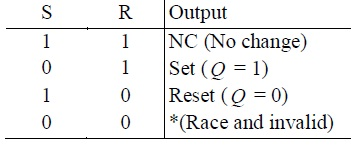Clocked RS Flip-flop:

Computers use thousands of flip-flops. To coordinate generally action, the square wave signal called the clock is sent to each flip-flop. Clock is applied to all flip-flops simultaneously; this makes sure that they all change states in unison. This synchronization is necessary in several digital systems.

In most of the synchronous systems output can change only when clock signal is making transition from 0 to 1, i.e. positive going transition (PGT) or 1 to 0, i.e. negative going transition (NGT). These systems are called as edge triggered. Symbols of edge triggered RS flip-flop that work with PGT and NGT are shown symbolically respectively.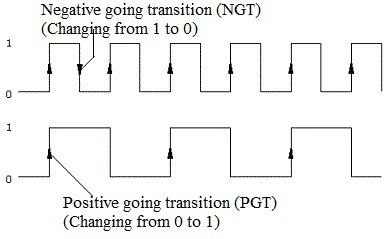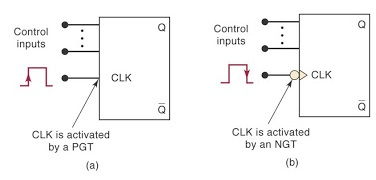The difference in symbol of clock activated by PGT and NOT. Change in control inputs R and S to flip-flop will not affect change in Q output until active clock (CLK) transition, i.e. the PGT in case of Figure (a) and a NOT in case of Figure (b), occurs.

Control inputs keep flip-flop ready to change and active clock transition at CLK input really triggers change. To make sure that clocked flip-flop responds correctly when active clock transition takes place, inputs should be stable, i.e., unchanging.

Consider circuit given below in which two extra NAND gates are utilized as clock pulse steering circuit and is triggered by PGT. A LOW (i.e. 0) clock CLK prevents S and R from controlling flip-flop, since with whatever values of S and R outputs of NAND-1 and NAND-2 will be 1 which won't generate any change in Q output of flip-flop.

Though, when CLK is HIGH (i.e. 1) and S = R = 0, outputs of two NAND gates will be 1 and there would be no change in Q output.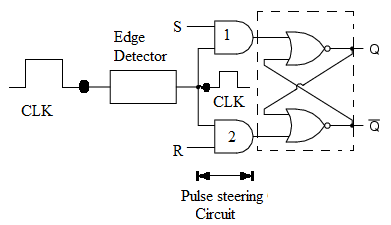The truth table for the positive edge triggered RS flip-flop. Q= Q0 is output level before arrival of PGT of the CLK. Arrow directed upward (↑) indicates that PGT is needed at the CLK.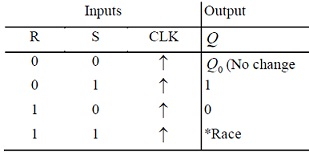Inputs S and R, and corresponding Q output, assuming initial value of Q, i.e., Q0, equal to 0. It is clear that at arrival of first clock transition both R and S are 0, thus there is no change in Q output that continues to be 0. But at arrival of second clock transition S is 1 and R is 0, this sets flip-flop with Q = 1 that doesn't change till third clock transition. At the time of third clock transition R is 1 and S = 0 that resets flip-flop with Q= 0. This is how Q output is traced. Between two PGTs of CLK, Q output doesn't change. It should be remembered, that whenever tracing the Q output corresponding to inputs, you have to look for active clock, note the values of inputs nand then decide value of Q output.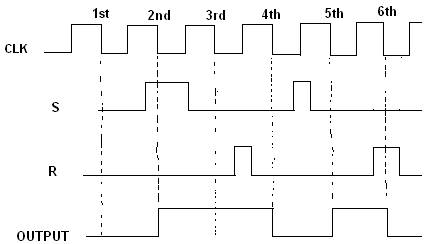The truth table of an RS flip-flop triggered by a NGT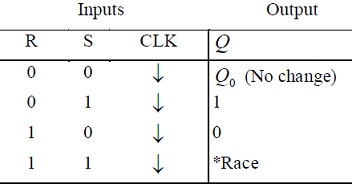PGT or NOT can be attained by using combination of gates or differentiating circuit comprising of capacitor and resistor.

Clocked D Flip-flop:

RS flip-flop has two inputs S and R. Producing two signals to drive the flip-flop is drawback in several applications. Also the race condition of both S and R low may take place inadvertently. To eliminate possibility of the race condition the new type of flip-flop is designed. This is known as a D flip-flop. Letter D stands for the data. The data input is given to S-input of RS flip-flop while same input goes to its R-input through the inverter. This symbol of edge triggered D flip-flop activated by the PGT. Its truth table shows that Q output of D flip-flop follows input data D. D input and corresponding Q output, assuming initial Q to be 1.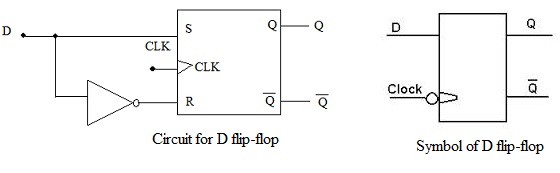Truth table for a positive edge triggered D flip-flop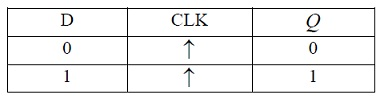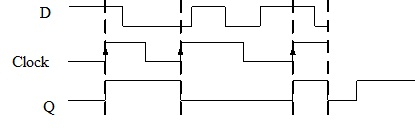D Latch:

At times edge trigger detecting circuit (such as RC combination) for D flip-flop is not utilized. In this case D flip-flop functions somewhat differently and is called as a D latch. Instead of edge triggering, level clock or ENABLE (abbreviated as EN) signal is utilized. When EN/CLK is 1, D will generate a 0 at either SET or CLEAR inputs of NAND latch to give a Q output to be at same level of D.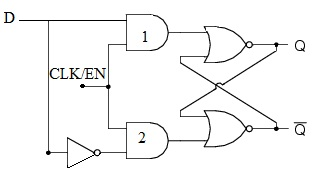When EN/CLK is 1, if D changes, Q will follow changes accurately like D as Q output doesn't have to wait for clock transition to respond to changes in D. D latch is therefore transparent to input in this mode. When EN/CLK is at 0, D is inhibited from affecting NAND latch as outputs of both steering NAND gates will be 1. Therefore Q and Q‾ continue to stay wherever they were before EN/CLK became 0. The outputs are latched to their current level and can't change during period EN/CLK is 0, even if D changes. The truth table of D latch is: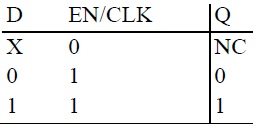Quite frequently two AND gates are introduced between pulse steering circuit and NAND. One input each of these AND gates are called as RESET (direct SET) and CLEAR (direct RESET) and are kept at 1 so as to permit output of pulse steering circuit to pass through. Though, if we want to set flip-flop irrespective of value of the D input, then we give a 0 to PRESET, that will set the flip-flop. Likewise, by giving a 0 to CLEAR will directly reset flip-flop. Symbol for D flip-flop with PRESET and CLEAR.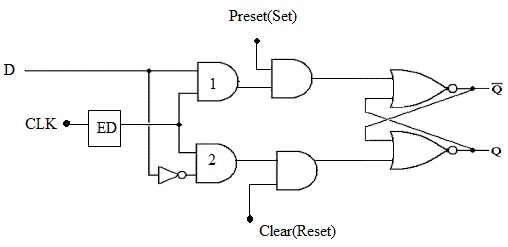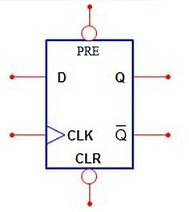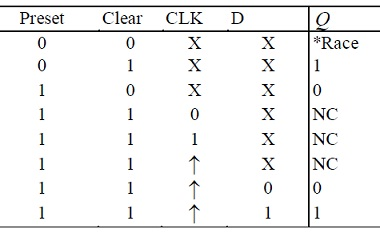Clocked JK Flip-flop:

When it comes to circuits that count, JK flip-flop is ideal element to use. The circuit and symbol for an edge triggered JK flip-flop is shown below.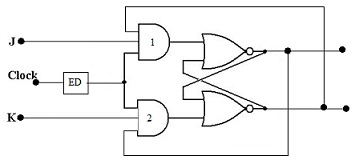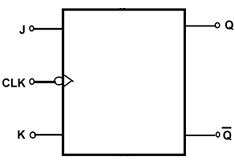Working of JK flip-flop is same as that of RS flip-flop except that race condition is absent. That is, there is no ambiguous result. Outputs Q and Q‾ of NAND latch are fed back to NAND-2 and NAND-1 respectively of pulse steering circuit that provides toggle operation. With J = K = 1, suppose that Q is 0 when clock transition arrives. With Q = 0 and Q‾ = 1, NAND-1 will steer PGT to set NAND latch to provide Q = 1. If we suppose Q= 1 when PGT of clock appears, NAND-2 will steer PGT to clear NAND latch to generate Q= 0. Therefore Q always ends up in opposite state. This is called as toggle mode of operation. If both J and K are left to state of 1, flip-flop will change state for each clock transition. Q output equal to Q0 signifies that new value of Q will be inverse of the value it had before PGT. Truth table of this flip-flop is given below and also J and K inputs and corresponding Q output.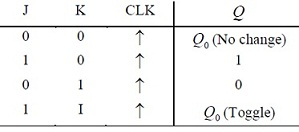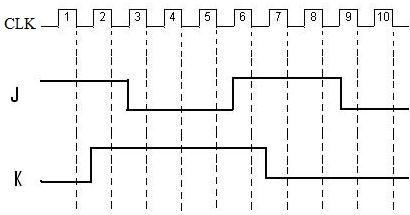Symbol for edge triggered JK flip-flop that is activated by NGT of the clock and its truth table is given below.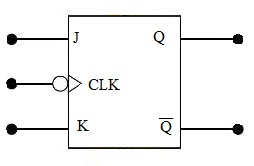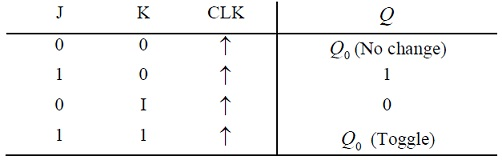Tutorsglobe: A way to secure high grade in your curriculum (Online Tutoring)

Expand your confidence, grow study skills and improve your grades.

Since 2009, Tutorsglobe has proactively helped millions of students to get better grades in school, college or university and score well in competitive tests with live, one-on-one online tutoring.

Using an advanced developed tutoring system providing little or no wait time, the students are connected on-demand with a tutor at www.tutorsglobe.com. Students work one-on-one, in real-time with a tutor, communicating and studying using a virtual whiteboard technology.  Scientific and mathematical notation, symbols, geometric figures, graphing and freehand drawing can be rendered quickly and easily in the advanced whiteboard.

Free to know our price and packages for online physics tutoring. Chat with us or submit request at info@tutorsglobe.com